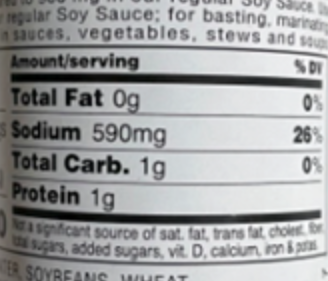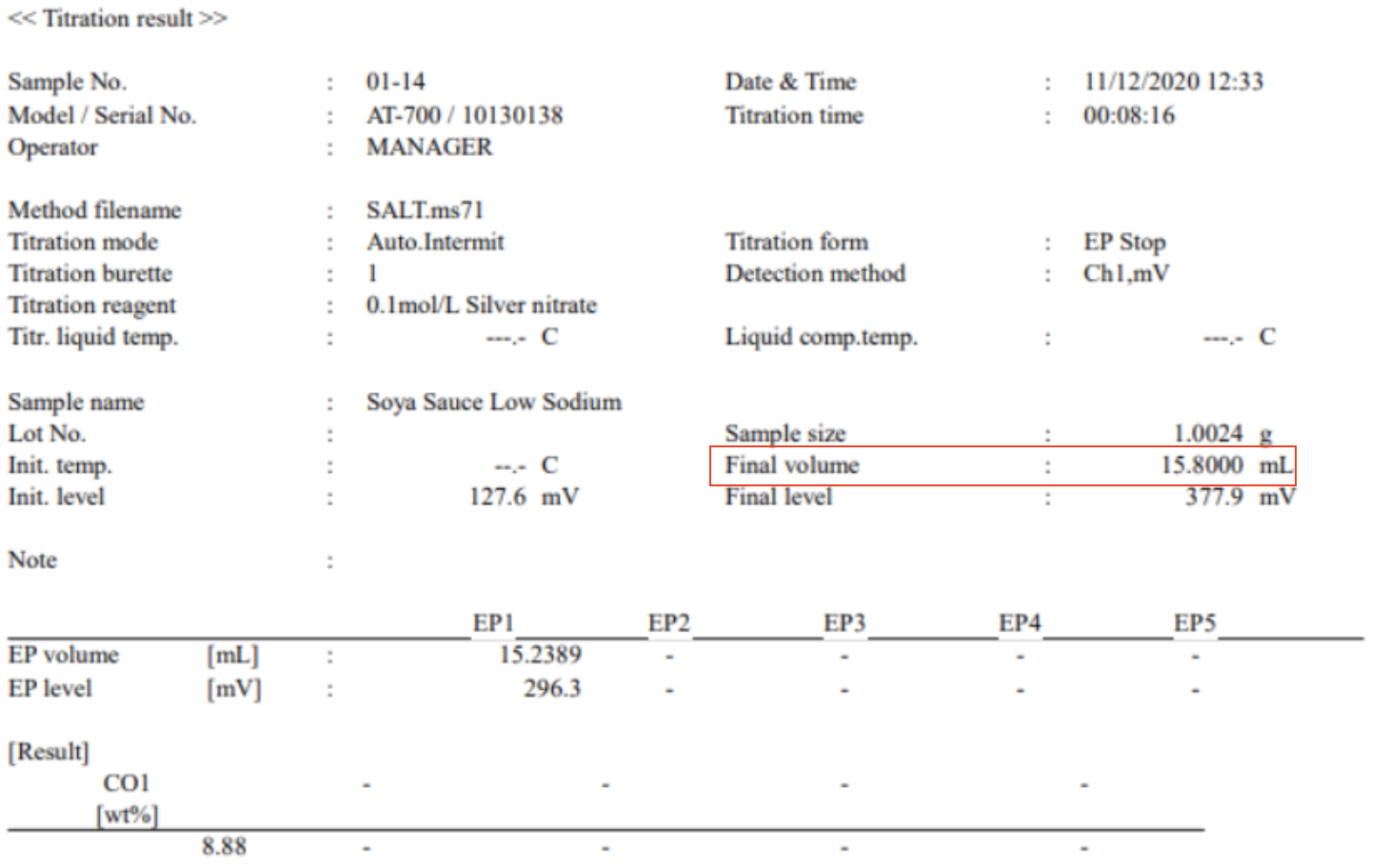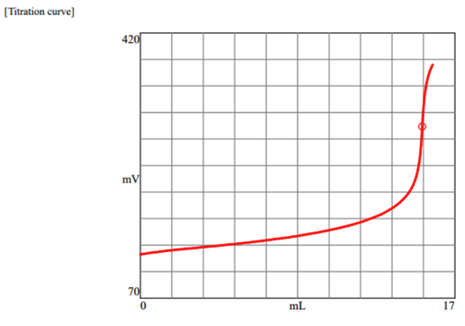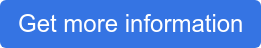## Salt Titration; How much does it cost per test?

We get this question a lot.  So, we decided to try and figure it out the best we can.  In this analysis, we are only going to examine and include the per-unit cost of the titrator (automatic) and the reagents (silver nitrate - AgNO3).  It seems we could include other factors including human resources and electrical power requirements but these can vary greatly so we will leave them out of this analysis.  Additional ancillary costs can always be added later.

Let's start off by assuming we purchase a basic automatic titrator for \$8,500.00

Next, let's examine our most required consumable.  Silver Nitrate- AgNO3.To do this we need to assume a sample with a given amount of sodium.  Sodium is generally expressed in mg on food labels.

We performed a salt titration on this food product (soy sauce).  On the label, we can see that the amount of sodium is 590mg of sodium per serving.  For this titration (see below), you can see we used 15.8ml of silver nitrate @ (0.1N mol).Note that silver nitrate can come in different strengths so there is a little math we need to consider when deciding on which strength of silver nitrate we should use.

Let's consider using a different strength silver nitrate.  Let's look at using 0.5N mol instead of the 0.1N mol we used in our test.  Initially, we used 0.1N for our titration.  So dividing 15.8mL by 5 helps us see that using a bit stronger silver nitrate will reduce our consumption of silver nitrate.  In this case,  around 3mL per test.   But it's not that simple.  Conversely, we can change the strength of the silver nitrate and make it stronger as long as you don't have other samples that have a lot less sodium.  Otherwise, it would be too strong for the other samples with less sodium and it would not be ideal in those situations (You probably would not find the endpoint).  Bottom line, if you are working with only one burette (doses the silver nitrate), then you are going to need to pick a silver nitrate strength that can be used for all of your samples.  The only alternative would be to refill the burette each time with different strength silver nitrate, or, have a swappable burette on hand.  Having a swappable burette means you can have preloaded burettes with different strength silver nitrate and you can then easily swap them on and off the titrator when needed.  Even better, some titrators can be configured to have two or more onboard burettes which would alleviate the need for manually swapping burettes.

Let's ignore this for the moment and continue to focus on our cost analysis assuming a single burette.

To give you an idea about pricing for silver nitrate.

•  We recently purchased some 0.1N mol Silver Nitrate (strength).  A 1 Liter bottle cost approximately \$75.00.  Divide \$75.00 by 1,000 and the per mL cost is \$0.075 per mL of 0.1N mol silver nitrate.   Assume 15.8mL of 0.1N mol silver nitrate per test => 15.8x\$0.075=\$1.19 per test.
• Assume 0.5N mol Silver Nitrate (strength). => 15.8mL divided by 5 = 3.2mL of silver nitrate per test. (e.g. you will use less with stronger).  Some recent pricing for 0.5N mol; \$170.00 / 500mL => \$0.34 per mL, or Bulk pricing if you buy a larger bottle \$1,000.00 / 4L (4,000mL) => \$0.25 per mL ~ 3.2mL per test x \$0.34 per mL = \$1.09 per test or 3.2mL x \$.25 per mL = \$0.80 per test.

So if you can purchase silver nitrate in bulk you can drive down your per test cost.

When you decide to test for salt (NaCl) using a titrator you are going to have that initial outlay to pay for the titrator.  After that, it's really a question of what strength of silver nitrate you are using and the amount you are using.   Hopefully, from this example, you can see how the price per test can range from about \$0.80 per test to almost \$1.20 per test.  So if we do some estimating you could say on average it costs about \$1.00 per test.  If you are testing 200 samples per day your daily cost for silver nitrate will cost you about \$200.00.

Now let's assume depreciation of the titrator using the straight-line method with the useful life of the titrator equal to 5 years (I think this is what a tax accountant would assume for tax purposes).  \$8,500 / 5 = \$1,700.00 per year.  Let's assume 251 working days per year ==> \$1,700 / 251 = \$6.73 per working day in depreciation expense.  In this case, divide that by 200 tests today, and that = \$0.03 per test.

I think these numbers (for the most part) provide a good working basis for determining daily operating costs.  They're probably not perfect but they show you how to go about figuring this out. As always we hope you find this information helpful!